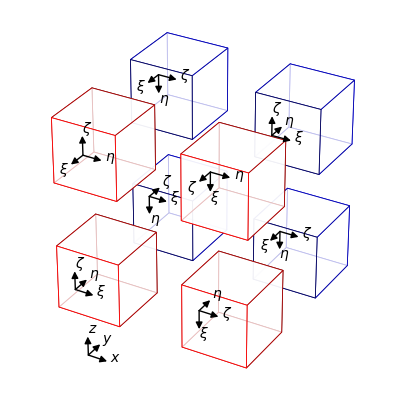domain::creators::RotatedBricks< TargetFrame > Class Template Reference

Create a 3D Domain consisting of eight rotated Blocks. More...

#include <RotatedBricks.hpp>

## Classes

struct  InitialGridPoints

struct  InitialRefinement

struct  IsPeriodicIn

struct  LowerBound

struct  Midpoint

struct  UpperBound

## Public Types

using options = tmpl::list< LowerBound, Midpoint, UpperBound, IsPeriodicIn, InitialRefinement, InitialGridPoints >Public Types inherited from DomainCreator< 3, TargetFrame >
using creatable_classes = typename DomainCreators_detail::domain_creators< VolumeDim >::template creators< TargetFrame >

## Public Member Functions

RotatedBricks (typename LowerBound::type lower_xyz, typename Midpoint::type midpoint_xyz, typename UpperBound::type upper_xyz, typename IsPeriodicIn::type is_periodic_in, typename InitialRefinement::type initial_refinement_level_xyz, typename InitialGridPoints::type initial_number_of_grid_points_in_xyz) noexcept

RotatedBricks (const RotatedBricks &)=delete

RotatedBricks (RotatedBricks &&) noexcept=default

RotatedBricksoperator= (const RotatedBricks &)=delete

RotatedBricksoperator= (RotatedBricks &&) noexcept=default

Domain< 3, TargetFrame > create_domain () const noexcept override

std::vector< std::array< size_t, 3 > > initial_extents () const noexcept override
Obtain the initial grid extents of the block with the given index.

std::vector< std::array< size_t, 3 > > initial_refinement_levels () const noexcept override
Obtain the initial refinement levels of the blocks.Public Member Functions inherited from DomainCreator< 3, TargetFrame >
DomainCreator (const DomainCreator< VolumeDim, TargetFrame > &)=delete

DomainCreator (DomainCreator< VolumeDim, TargetFrame > &&) noexcept=default

DomainCreator< VolumeDim, TargetFrame > & operator= (const DomainCreator< VolumeDim, TargetFrame > &)=delete

DomainCreator< VolumeDim, TargetFrame > & operator= (DomainCreator< VolumeDim, TargetFrame > &&) noexcept=default

## Static Public Attributes

static constexpr OptionString help

## Detailed Description

### template<typename TargetFrame> class domain::creators::RotatedBricks< TargetFrame >

Create a 3D Domain consisting of eight rotated Blocks.The orientations of the blocks are described in two different ways:

• 1 - As orientations of blocks relative to the physical cartesian axes.
• 2 - As rotations relative to the physical cartesian axes written using Rubik's cube rotation notation, for the sake of shorter variable names.

For reference, this is the notation used:

• U - A clockwise 90 degree rotation about the +z axis (The "up" side)
• R - A clockwise 90 degree rotation about the +x axis (The "right" side)
• F - A clockwise 90 degree rotation about the -y axis (The "front" side)

For reference, D, L, and B ("down", "left", and "back") are the inverse rotation to the aforementioned ones, respectively. Note: Whereas Rubik's cube rotations rotate a layer of the 3x3 puzzle cube, we are adopting the notation to apply to rotations of the cube itself.

• The -x, -y, -z block has the aligned orientation, that is, xi is aligned with x, eta is aligned with y, and zeta with z.
• The +x, -y, -z block has the orientation (zeta, eta, -xi). It corresponds to the orientation obtained by the rotation F.
• The -x, +y, -z block has the orientation (xi, zeta, -eta). It corresponds to the orientation obtained by the rotation R.
• The +x, +y, -z block has the orientation (zeta, -xi, -eta). It corresponds to the orientation obtained by the rotation F followed by the rotation R.
• The -x, -y, +z block has the orientation (eta, -xi, zeta). It corresponds to the orientation obtained by the rotation U.
• The +x, -y, +z block has the orientation (eta, -zeta, -xi). It corresponds to the orientation obtained by the rotation F followed by the rotation U.
• The -x, +y, +z block has the orientation (zeta, -xi, -eta). It corresponds to the orientation obtained by the rotation R followed by the rotation U (equivalently, F followed by R).
• The +x, +y, +z block also has the aligned orientation (xi, eta, zeta), relative to the edifice. It is not aligned relative to its neighbors.

This DomainCreator is useful for testing code that deals with unaligned blocks.

## ◆ help

template<typename TargetFrame >
 constexpr OptionString domain::creators::RotatedBricks< TargetFrame >::help
static
Initial value:
= {
"A DomainCreator useful for testing purposes.\n"
"RotatedBricks uses eight rotated Blocks to create the rectangular\n"
"prism [LowerX,UpperX] x [LowerY,UpperY] x [LowerZ,UpperZ]. The\n"
"outermost index to InitialGridPoints is the dimension index, and\n"
"the innermost index is the block index along that dimension."}

The documentation for this class was generated from the following files: